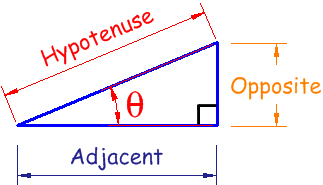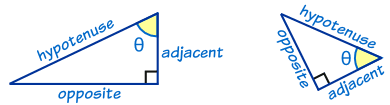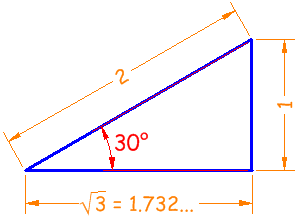# Naija Google Blogger

Naija we hail oooo Una no dey fail oooo even when the country they wail lli oooo

## Connect With Us

##### Email Newsletter

Subscribe to our newsletter to get the latest updates to your inbox. ;-)

Your email address is safe with us!

## Right Triangle

OK, let's see what this is all about.

Firstly, the names Opposite, Adjacent and Hypotenuse come from the right triangle:• "Opposite" is opposite to the angle θ
• "Adjacent" is adjacent (next to) to the angle θ
• "Hypotenuse" is the long oneAdjacent is always next to the angle
(and opposite is opposite the angle)

## Sine, Cosine and Tangent

And Sine, Cosine and Tangent are the three main functions in trigonometry.

They are often shortened to sin, cos and tan.

The calculation is simply one side of a right angled triangle divided by another side ... we just have to know which sides, and that is where "sohcahtoa" helps.

For a triangle with an angle θ , the functions are calculated this way:

 Sine Function: soh... sin(θ) = opposite / hypotenuse Cosine Function: ...cah... cos(θ) = adjacent / hypotenuse Tangent Function: ...toa tan(θ) = opposite / adjacent

### Example: what are the sine, cosine and tangent of 30° ?

The classic 30° triangle has a hypotenuse (the long side) of length 2, an opposite side of length 1 and an adjacent side of √3, like this:Now we know the lengths, we can calculate the functions:

 Sine soh... sin(30°) = 1 / 2 = 0.5 Cosine ...cah... cos(30°) = 1.732 / 2 = 0.866 Tangent ...toa tan(30°) = 1 / 1.732 = 0.577

(get your calculator out and check them!)

## How to Remember

Well, "sohcahtoa" may be easy for you to remember ... but here's another way to help it:

Sailors Often Have Curly Auburn Hair Till Old Age.

Or perhaps you prefer one of these:

• Some Old Horses Can Always Hear Their Owners Approach.
• Some Old Hen Caught Another Hen Taking One Away.
--

## Entertainment

##### Subscribe Via Email

Subscribe to our newsletter to get the latest updates to your inbox. ;-)

Your email address is safe with us!

(C) Copyright by Naija Google Blogger
Wordpress Theme by QualityWP | Conveted to Blogger Template by ThemeLib.com

## About MeLearn More →

# Trigonometry Right Angle Triangle {SOHCAHTOA}

## Right Triangle

OK, let's see what this is all about.

Firstly, the names Opposite, Adjacent and Hypotenuse come from the right triangle:• "Opposite" is opposite to the angle θ
• "Adjacent" is adjacent (next to) to the angle θ
• "Hypotenuse" is the long oneAdjacent is always next to the angle
(and opposite is opposite the angle)

## Sine, Cosine and Tangent

And Sine, Cosine and Tangent are the three main functions in trigonometry.

They are often shortened to sin, cos and tan.

The calculation is simply one side of a right angled triangle divided by another side ... we just have to know which sides, and that is where "sohcahtoa" helps.

For a triangle with an angle θ , the functions are calculated this way:

 Sine Function: soh... sin(θ) = opposite / hypotenuse Cosine Function: ...cah... cos(θ) = adjacent / hypotenuse Tangent Function: ...toa tan(θ) = opposite / adjacent

### Example: what are the sine, cosine and tangent of 30° ?

The classic 30° triangle has a hypotenuse (the long side) of length 2, an opposite side of length 1 and an adjacent side of √3, like this:Now we know the lengths, we can calculate the functions:

 Sine soh... sin(30°) = 1 / 2 = 0.5 Cosine ...cah... cos(30°) = 1.732 / 2 = 0.866 Tangent ...toa tan(30°) = 1 / 1.732 = 0.577

(get your calculator out and check them!)

## How to Remember

Well, "sohcahtoa" may be easy for you to remember ... but here's another way to help it:

Sailors Often Have Curly Auburn Hair Till Old Age.

Or perhaps you prefer one of these:

• Some Old Horses Can Always Hear Their Owners Approach.
• Some Old Hen Caught Another Hen Taking One Away.
--

### About Naija Google Blogger

This is a short description in the author block about the author. You edit it by entering text in the "Biographical Info" field in the user admin panel.